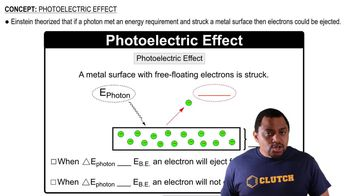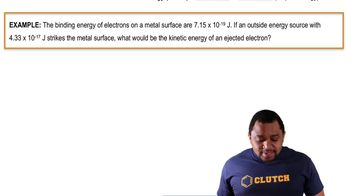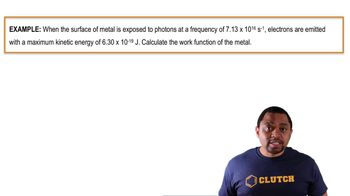Start typing, then use the up and down arrows to select an option from the list.# Photoelectric Effect Example 2

Jules Bruno
170views
3
here. It says what? The surface of the metal exposed of photons at a frequency of 7.13 times to the 16 seconds inverse electrons air admitted with a maximum kinetic energy of 6.30 times 10 to the negative 19 jewels. Here, we need to calculate the work function off the metal. Alright, So first of all, remember, work function is another name for binding energy or threshold frequency. So this is E. Now, remember, we're gonna say the energy total energy of our photon equals binding energy or, in this case, work function, plus kinetic energy in terms of energy of a photon that equals plank's constant times your frequency. So using that frequency given to us initially will help us to figure out the total energy of our photon. So here those plank's constant plug in your frequency. Okay, so when we do that, we're gonna get our energy of our photon as 4.7243 times 10 to the negative 17 jewels. So take that and plug it in okay for three times 10 to the negative 17 jewels binding energy. Or, in this case, work function is what we're looking for. And they give us the kinetic energy as 6.30 times 10 to the negative 19 jewels. Subtract that out from both sides and we'll have our work function at the end. So then here, when we take that and plug it in, we're gonna have our binding energy or our work function as equaling 4.66 times 10 to the negative jewels as our final answer for our01:2601:1101:2201:1801:52